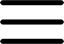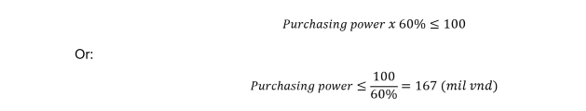Margin requirement

Concept: Margin ratio is the ratio between the real assets value to the total assets on the margin trading account. There are 2 types of margin ratios: Initial Margin Rate and Margin. Currently the Minimum Initial Margin Rate at Pinetree is 50%, Maintenance Margin Rate is 40%.

Initial margin ratio (IMR) is the ratio of the real asset value to the value of securities expected to be bought by margin trading orders calculated according to the market price at the time of the transaction.

Example 1

You have 100 million VND in cash on your account and want to buy stock X with the loan ratio of 40% (equivalent to the initial margin ratio: 100% – 40% = 60%). At that time, the value of stock X you are eligible to buy on the margin account is calculated as follows:This means that you can buy up to VND 167 million of stock X with your margin buying power.

Maintenance Margin Ratio (MMR) is the minimum ratio of real assets to the total asset value on a margin trading account. This ratio is set to assist investors in reducing risks in the face of market fluctuations.

Example 2

You have 200 million VND of stock X bought with 100 million VND of equity capital and 100 million VND of margin loan from Pinetree. Due to the price decrease, the value of stock X on your account is only VND 150 million VND. Margin loan from Pinetree is still 100 million VND, so your equity is only 50 million VND. The margin rate on your account is 33% (as the formula below), which is lower than maintenance margin rate)In this case, the client should replenish assets so that the margin rate increases to the maintenance margin rate to ensure the safety of the account.